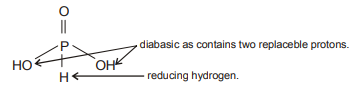# NEET Chemistry The p-Block Elements (XII) Questions Solved

For ${H}_{3}P{O}_{3}$ and ${H}_{3}P{O}_{4}$ the correct choice is :

(A) ${H}_{3}P{O}_{3}$  is diabasic and reducing agent.

(B) ${H}_{3}P{O}_{3}$  is diabasic and non-reducing agent.

(C) ${H}_{3}P{O}_{4}$  is tribasic and reducing agent.

(D) ${H}_{3}P{O}_{4}$  is tribasic and non- reducing agent.

(A)Oxidation state of P is + 3 can be decrease to – 3 and increase to + 5. Hence it can act as both
reducing as well as oxidising agent.

Difficulty Level:

• 48%
• 21%
• 12%
• 21%